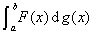[定义设在区间[a,b]上给定两个有界函数f(x)g(x).用任意方法把区间[a,b]分成若干部分，其分点为

a=x0<x1<x2<< xi<xi+1<<xn=b

σ=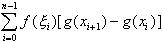λ0时，如果极限存在，那末这个极限称为函数f(x)对函数g(x)的斯蒂尔吉斯积分，记作特别是，当函数g(x)在区间上连续可微时，函数f(x)g(x)的斯蒂尔吉斯积分就是通常的黎曼积分[可积性]

1°若函数f(x)连续，函数g(x)有有界变差，则积分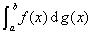1

2°若函数f(x)在区间[a,b]上黎曼可积，函数g(x)满足李普希茨条件：

|g(x')-g(x'')|L(x'x'')

(L为常数，ax''<x'b)

3°若函数f(x)在区间[a,b]上黎曼可积，函数g(x)可表示成

g(x)=C+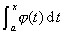[积分法则与不等式]

1°积分法则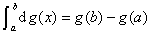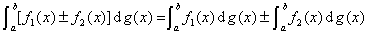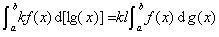(k,l为常数)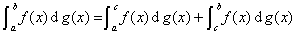(a<c<b,三个积分都存在，当上式右边两个积分存在时，一般不能推出积分存在）(分部积分公式)

2° g(x)在区间[a,b]上为一非减函数，则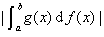3° g(x)在区间[a,b]上为一非减函数，则f(x)F(x),